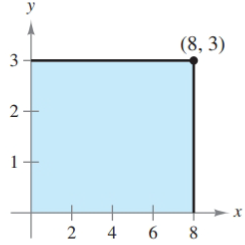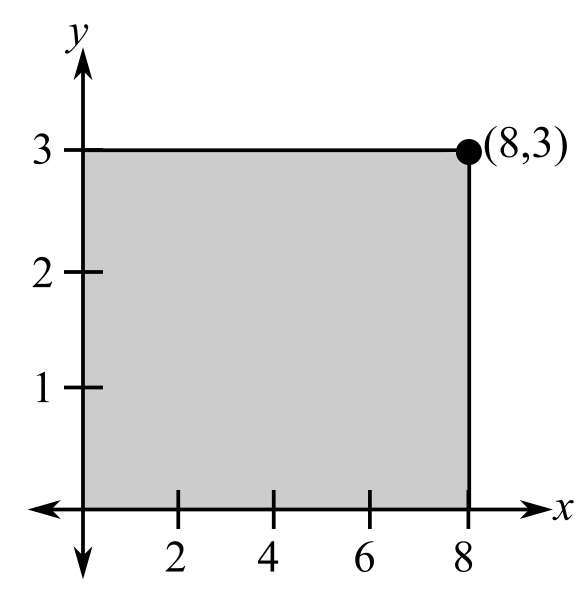Chapter 7.8, Problem 25E### Calculus: An Applied Approach (Min...

10th Edition
Ron Larson
ISBN: 9781305860919

#### Solutions

Chapter
Section### Calculus: An Applied Approach (Min...

10th Edition
Ron Larson
ISBN: 9781305860919
Textbook Problem
1 views

# Finding Area with a Double Integral In Exercises 25-30, use a double integral to find the area of the specified region. See Example 3.To determine

To calculate: The area of region by using double integration shown below.Explanation

Given Information:

The provided region is.

Formula used:

If a region is R defined in the domain of ayb and cxd, then,

The area of the region R is,

A=cdabdydx

Calculation:

Consider the region,

The bound for x are 0x8 and the bound for y are 0y3.

The area of the region is

A=0803dydx

Integrate with respect to y by holding x constant,

0803dydx=

### Still sussing out bartleby?

Check out a sample textbook solution.

See a sample solution

#### The Solution to Your Study Problems

Bartleby provides explanations to thousands of textbook problems written by our experts, many with advanced degrees!

Get Started

#### In Exercises 58, evaluate the expression. 7. |2 6|

Applied Calculus for the Managerial, Life, and Social Sciences: A Brief Approach

#### In problems 23-58, perform the indicated operations and simplify. 24.

Mathematical Applications for the Management, Life, and Social Sciences

#### True or False: n=1(1)nnn+3 converges.

Study Guide for Stewart's Single Variable Calculus: Early Transcendentals, 8th